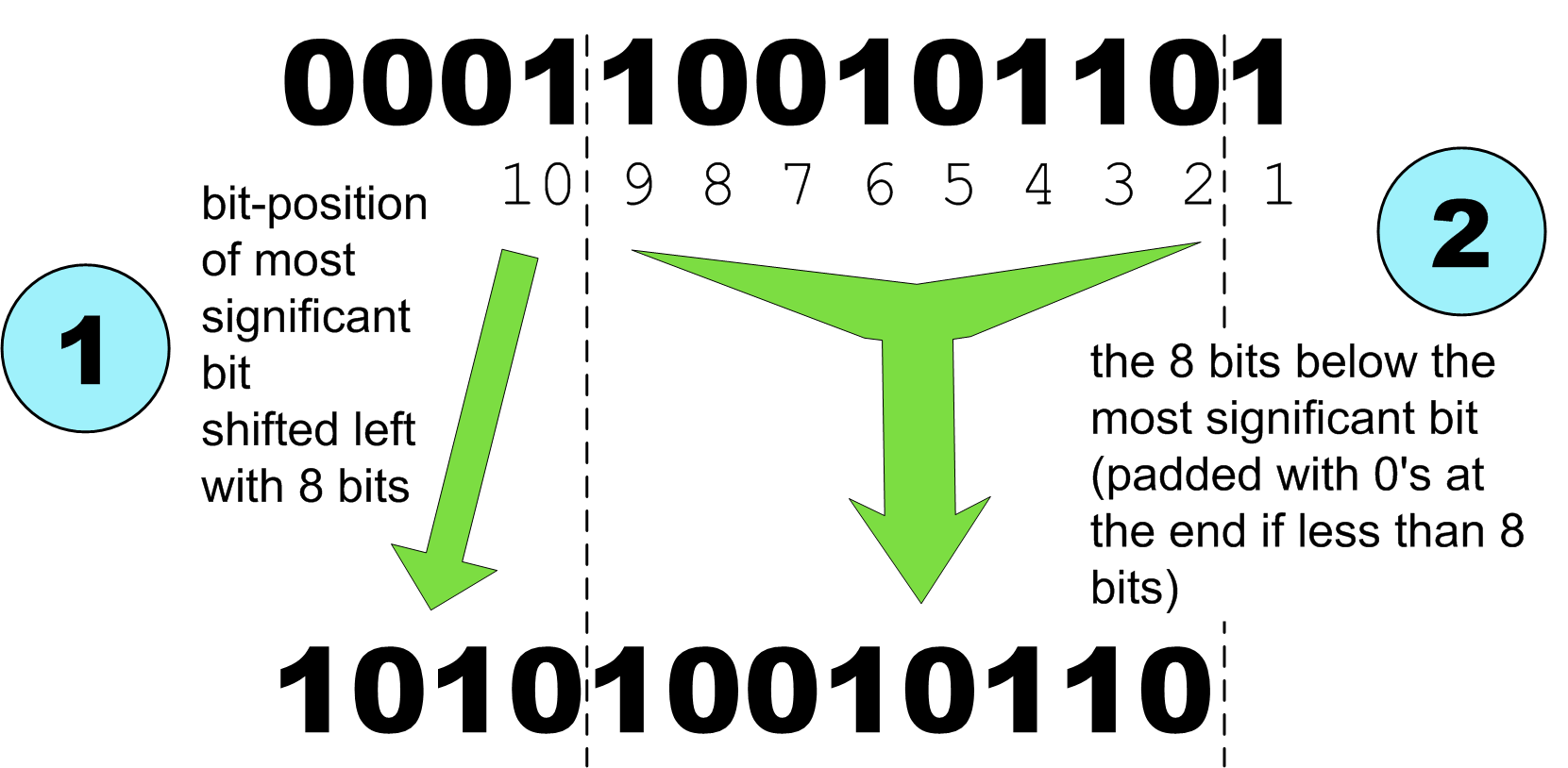#Forth-eV Wiki

pfw:2log

### 2LOG, calculate binary logarithm

The 2LOG routine was the result of the need for converting a linear input into something with a more logarithmic character. There was no need for high accuracy, but rather a short reliable routine. The routine uses a very simple principle, but the result is surprisingly useable. A prime example where focussing on the essentials results in an excellent solution.

The 2LOG routine produces as output a fixed floating point number with 8 bits after the decimal point. ( x…x.xxxxxxxx ) The number of bits before the decimal point depends on the native width of the stack.

#### The principle used is this:

looking at the number to be converted in binary representation: (see grafic below) - step 1: take the bit-position+1 of the first set bit as number before the decimal point - step 2: as 8 bit fraction take the highest 8 bits following the most most significant set bit. If there are less than 8 bits, pad the end with cleared bits up to 8 bits.

It is good to notice that the fractional part forms a linear interpolation between two consecutive log numbers. For most purposes that is accurate enough.#### the generic Forth program

As example we look at the routine for a 16b Forth. The other example is suitable for all Forth implementations.

```decimal
: 2LOG16b ( u -- y )
16 0 do
s>d if
2* 8 rshift                 \ linear interpolation
15 i -                      \ logarithmic class
8 lshift or leave
then 2*
loop ;```
• The programs does maximal 16 loops.
• For each loop S>D is used to check if the most significant bit is set. If not, then the program shifts the number 1 bit to the left with 2*.
• Otherwise it calculates the output.
• The logarithmic part is calculated by subtracting the index from 15.
• The fractional part is calculated by shifting to left with 1bit, followed by shifting 8 bits to the right.
• Finally, both numbers are then combined into one number with a shift and or as final output.

#### The general version

The general version is suitable for all Forth-implementations which have a multiple of 8 bits as cell-width. It functions in exactly the same way as the 16b example above. But during compilation it calculates the, for that Forth-implementation relevant, values for the doloop, shift and subtraction.

```: 2LOG ( u -- y )
[ 8 cells ] literal 0 do                \ #bits/cell
s>d if  2*
[ 8 cells 8 - ] literal rshift  \ linear interpolation
[ 8 cells 1- ]  literal  i -    \ logarithmic class
8 lshift or leave
then 2*
loop ;```

## Alternative Implementations

pfw/2log.txt · Zuletzt geändert: 2023-02-27 18:41 von uho

### Seiten-Werkzeuge